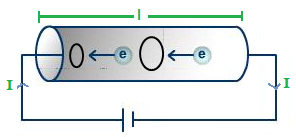# Mechanism of metallic conductor, Drift velocity and Current

A metal consist of free electrons due to the thermal energy .they are moving randomly in all direction by colliding with their neighbouring atoms or ions, but they don’t display in particular direction. Thus these electrons do not produce electric current.When connect a conductor with a battery, electric field will be developed,within the conductor in the direction of conventional current .Then the electrons will experience force. Thus, the electrons get displace in the opposite direction of the electrons field. Thus current is produced in the conductor

Drift velocity: The average velocity of the electrons in the opposite direction of the electric field is called drift velocity.

Let us consider a metallic conductor of length ‘l’ cross-section area ‘A’ is connected with a battery as shown in figure.

Now, volume of the conductor(V)=A × l

Let ‘N ‘be the total no of electrons per unit volume and ‘n’ be the number of electron per unit volume.

Then,

$\text{n} = \frac{\text{N}}{ \text{V}}$

or, N =n.V

or,N =n.A.l (∵ V = A × l )

let ‘e’ be the charge of an electron

Then, total charge of an electron (q)= N.e

or, q = N.A.l.e

Now, electric current (I) =  $\frac{\text{q}}{ \text{t}}$

or, $\text{I} = \frac{\text{n.A.l.e}}{ \text{t}}$

or, I= nAve

∴ I = venA

Current Density (D): The amount of current per unit cross sectional area and perpendicular to the surface is called Current Density.

i.e J=   $\frac{\text{I}}{ \text{A}}$

or, $\text{I} = \frac{\text{v.e.n.A}}{ \text{A}}$

$\text{∴ J = Ven}$

This is the relation between electric current density.

Do you like this article ? If yes then like otherwise dislike : 4